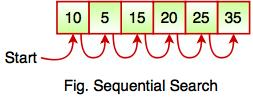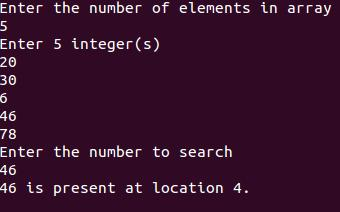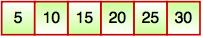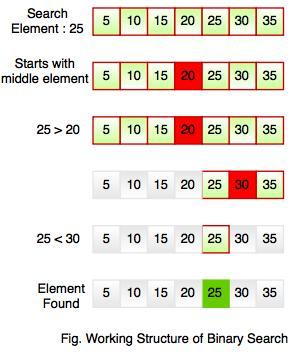# Searching in Data Structure

## What is Searching?

• Searching is the process of finding a given value position in a list of values.
• It decides whether a search key is present in the data or not.
• It is the algorithmic process of finding a particular item in a collection of items.
• It can be done on internal data structure or on external data structure.

## Searching Techniques

To search an element in a given array, it can be done in following ways:

1. Sequential Search
2. Binary Search

#### 1. Sequential Search

• Sequential search is also called as Linear Search.
• Sequential search starts at the beginning of the list and checks every element of the list.
• It is a basic and simple search algorithm.
• Sequential search compares the element with all the other elements given in the list. If the element is matched, it returns the value index, else it returns -1.The above figure shows how sequential search works. It searches an element or value from an array till the desired element or value is not found. If we search the element 25, it will go step by step in a sequence order. It searches in a sequence order. Sequential search is applied on the unsorted or unordered list when there are fewer elements in a list.

The following code snippet shows the sequential search operation:

function searchValue(value, target)
{
for (var i = 0; i < value.length; i++)
{
if (value[i] == target)
{
return i;
}
}
return -1;
}
searchValue([10, 5, 15, 20, 25, 35] , 25);   // Call the function with array and number to be searched

#### Example: Program for Sequential Search

#include <stdio.h>
int main()
{
int arr, search, cnt, num;

printf("Enter the number of elements in array\n");
scanf("%d",&num);

printf("Enter %d integer(s)\n", num);

for (cnt = 0; cnt < num; cnt++)
scanf("%d", &arr[cnt]);

printf("Enter the number to search\n");
scanf("%d", &search);

for (cnt = 0; cnt < num; cnt++)
{
if (arr[cnt] == search)     /* if required element found */
{
printf("%d is present at location %d.\n", search, cnt+1);
break;
}
}
if (cnt == num)
printf("%d is not present in array.\n", search);

return 0;
}

Output:#### 2. Binary Search

• Binary Search is used for searching an element in a sorted array.
• It is a fast search algorithm with run-time complexity of O(log n).
• Binary search works on the principle of divide and conquer.
• This searching technique looks for a particular element by comparing the middle most element of the collection.
• It is useful when there are large number of elements in an array.

•• The above array is sorted in ascending order. As we know binary search is applied on sorted lists only for fast searching.
For example, if searching an element 25 in the 7-element array, following figure shows how binary search works:Binary searching starts with middle element. If the element is equal to the element that we are searching then return true. If the element is less than then move to the right of the list or if the element is greater than then move to the left of the list. Repeat this, till you find an element.

#### Example: Program for Binary Search

#include<stdio.h>
#include<conio.h>

void main()
{
int f, l, m, size, i, sElement, list; //int f, l ,m : First, Last, Middle
clrscr();

printf("Enter the size of the list: ");
scanf("%d",&size);

printf("Enter %d integer values : \n", size);

for (i = 0; i < size; i++)
scanf("%d",&list[i]);

printf("Enter value to be search: ");
scanf("%d", &sElement);

f = 0;
l = size - 1;
m = (f+l)/2;

while (f <= l) {
if (list[m] < sElement)
f = m + 1;
else if (list[m] == sElement) {
printf("Element found at index %d.\n",m);
break;
}
else
l = m - 1;
m = (f + l)/2;
}
if (f > l)